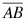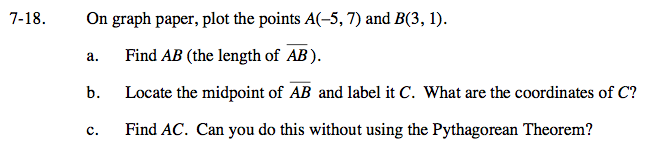### Home > GC > Chapter 7 > Lesson 7.1.2 > Problem7-18

7-18.
1. On graph paper, plot the points A(−5, 7) and B(3, 1). 7-18 HW eTool (Desmos). Homework Help ✎

1. Find AB (the length of).

2. Locate the midpoint ofand label it C. What are the coordinates of C?

3. Find AC. Can you do this without using the Pythagorean Theorem?Use the Pythagorean Theorem to find the length of AB.

Use two congruent slope triangles to find the midpoint.

Because C is the midpoint, what does that tell you about the length of AC?

Use the eTool below to find the length and midpoint of AB.
Click the link at right for the full version of the eTool: GC 7-18 HW eTool